Function Repository Resource:

# ImageGeoLocation

Find the location at which a picture was taken

Contributed by: Giulio Alessandrini
 ResourceFunction["ImageGeoLocation"][img] returns the estimated GeoLocation[…] at which img was taken. ResourceFunction["ImageGeoLocation"][img,n] returns the first n probable locations. ResourceFunction["ImageGeoLocation"][img,n,"Probabilities"] returns the locations together with their probabilities. ResourceFunction["ImageGeoLocation"][{img1,img2,…},…] estimates multiple locations at once.

## Details

ImageGeoLocation uses the neural network ResNet-101 to determine probable locations.

## Examples

### Basic Examples (2)

Find the location at which a picture was taken:

 In:=Out=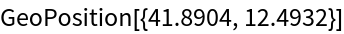Find multiple location candidates:

 In:=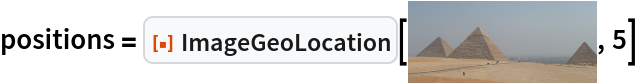Out=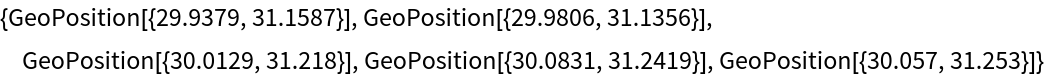Map them:

 In:=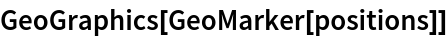Out=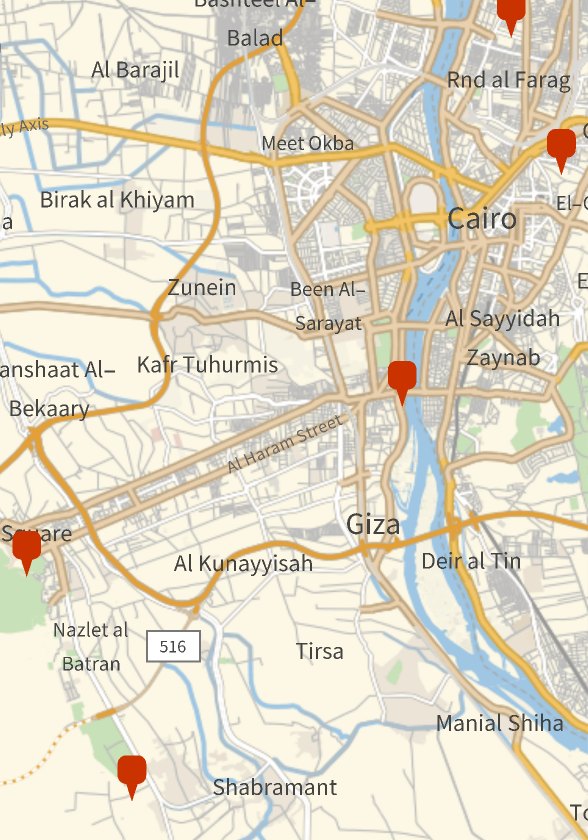### Scope (5)

Predict one location:

 In:=Out=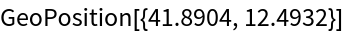Return the first n most probable locations:

 In:=Out=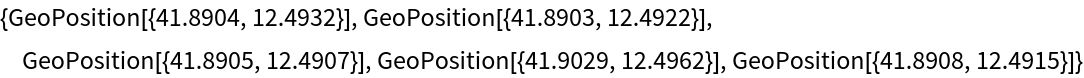Predict locations for multiple images:

 In:=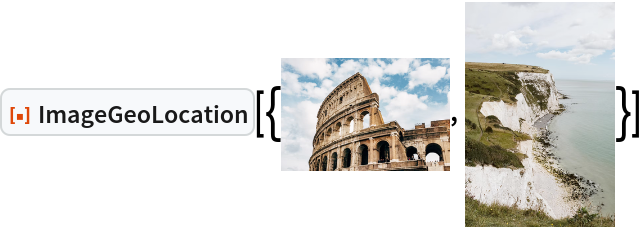Out=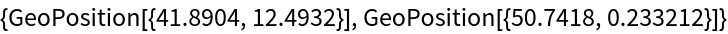Return the first n most probable locations for multiple images:

 In:=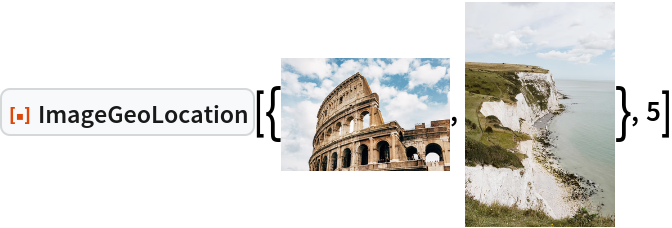Out=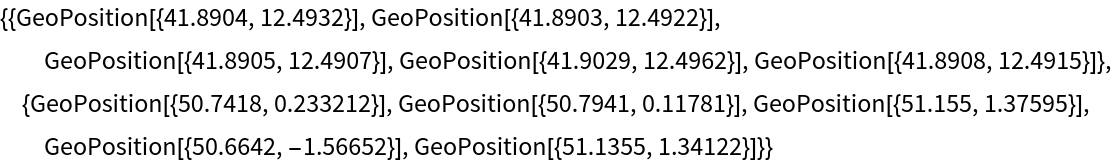Return locations together with their probabilities:

 In:=Out=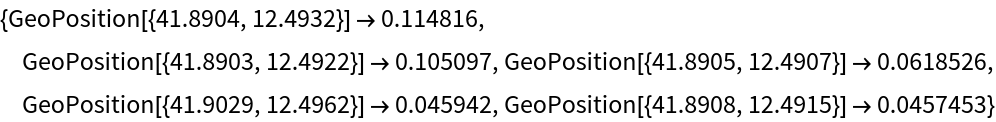In:=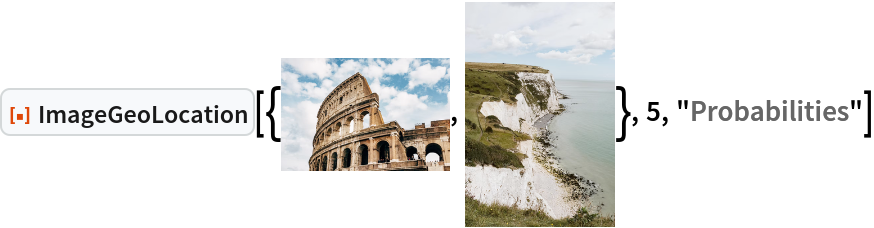Out=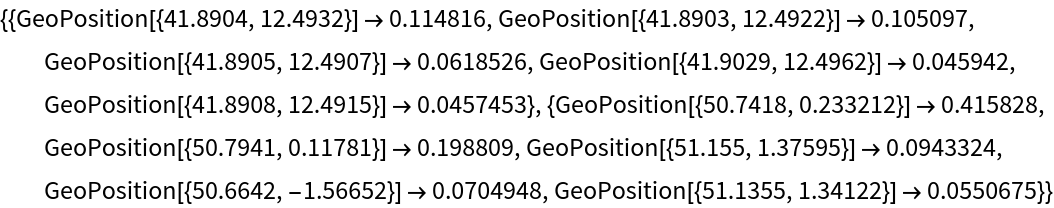### Applications (2)

In places with high population density, very fine-grained predictions are possible. Consider the following four landmarks in Paris:

 In:=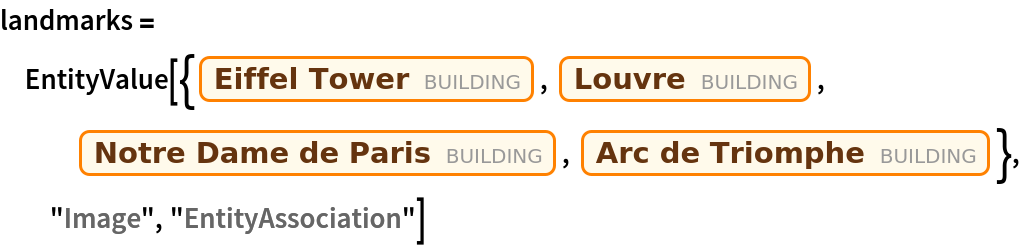Out=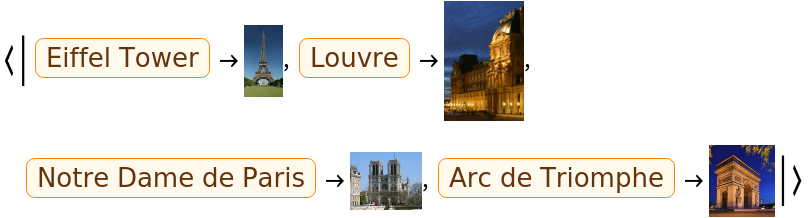Predict the location of the four landmarks:

 In:=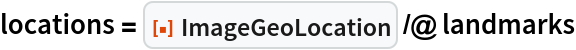Out=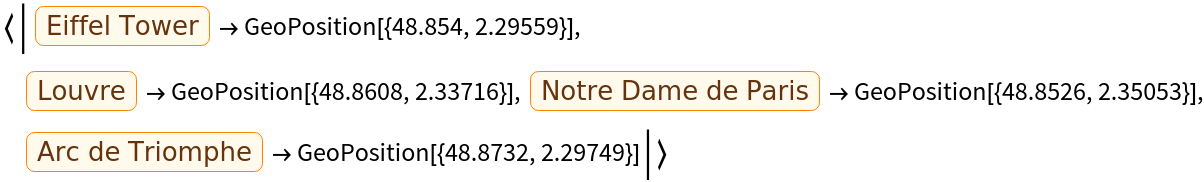Plot the locations on the map:

 In:=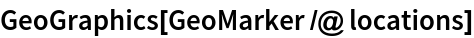Out=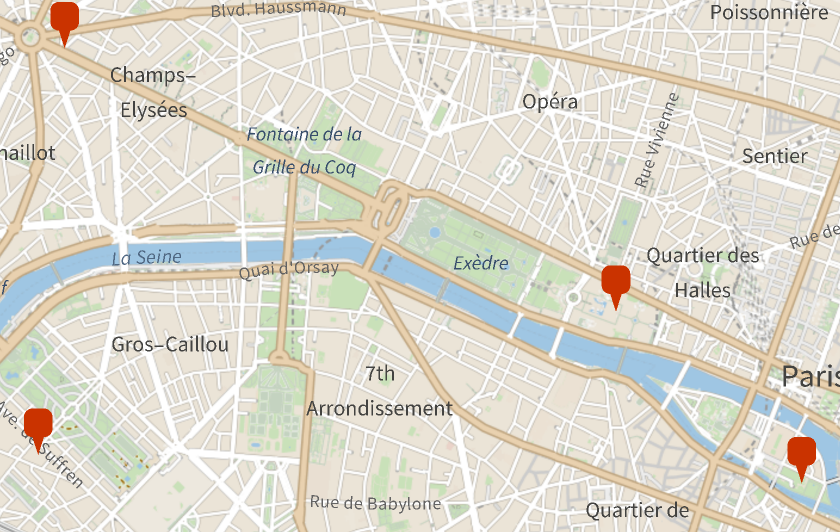Compare to the locations from Wolfram|Alpha:

 In:=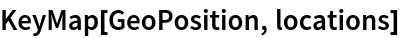Out=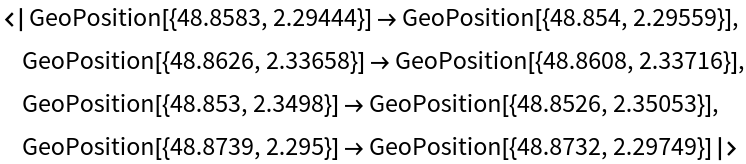Find the most probable locations for an image of white cliffs:

 In:=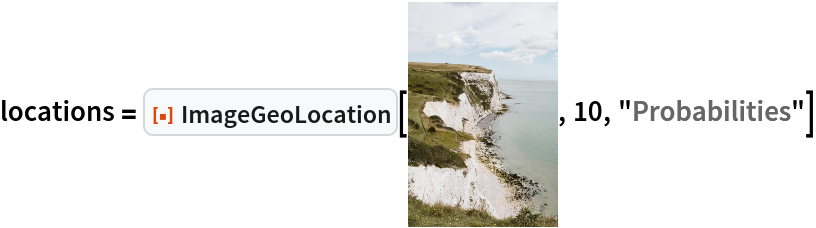Out=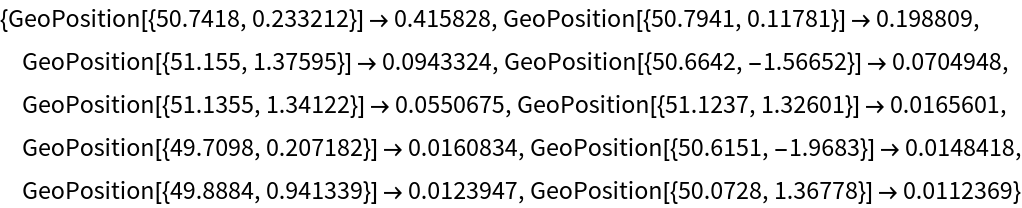Plot them in a bubble chart where the radius is given by the probability value:

 In:=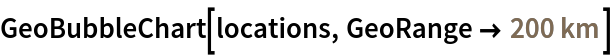Out=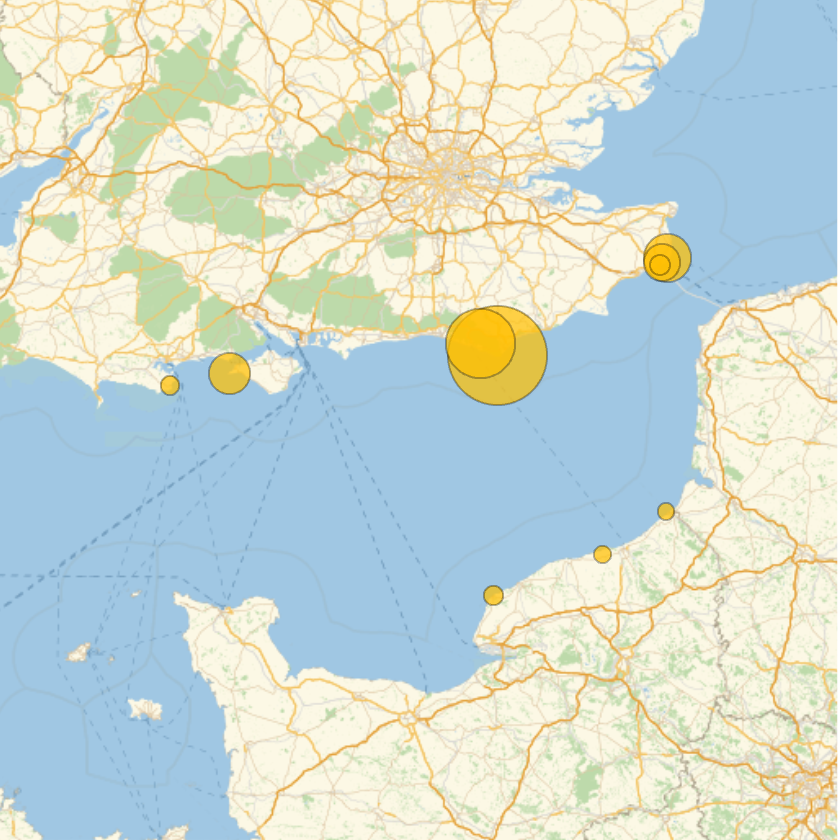## Version History

• 1.0.0 – 25 January 2023